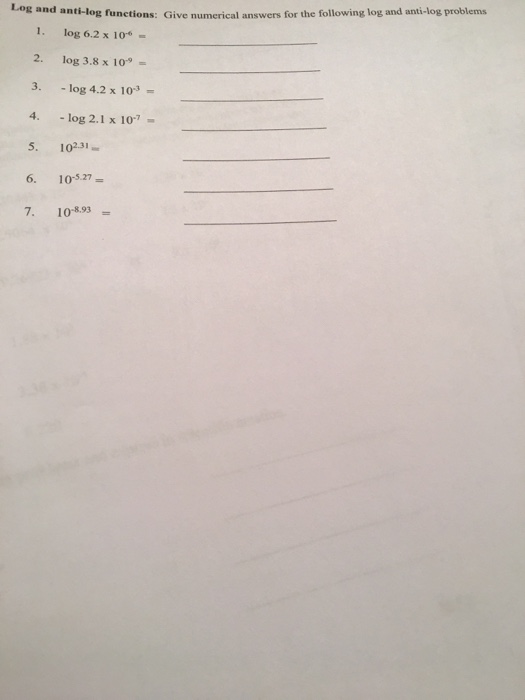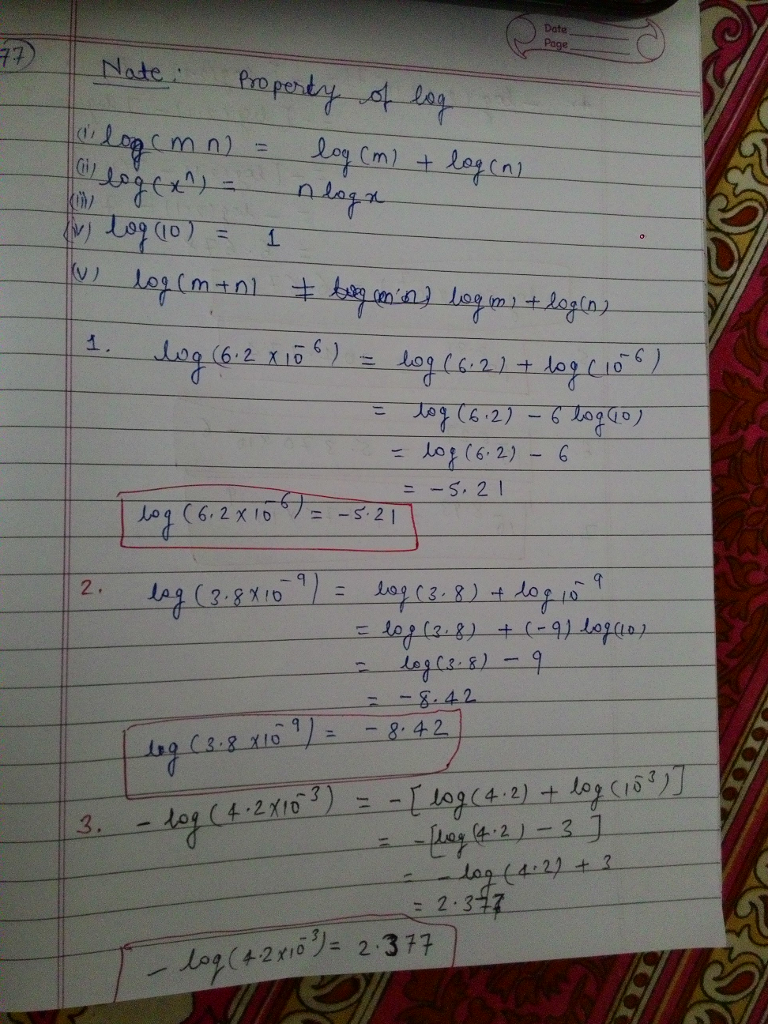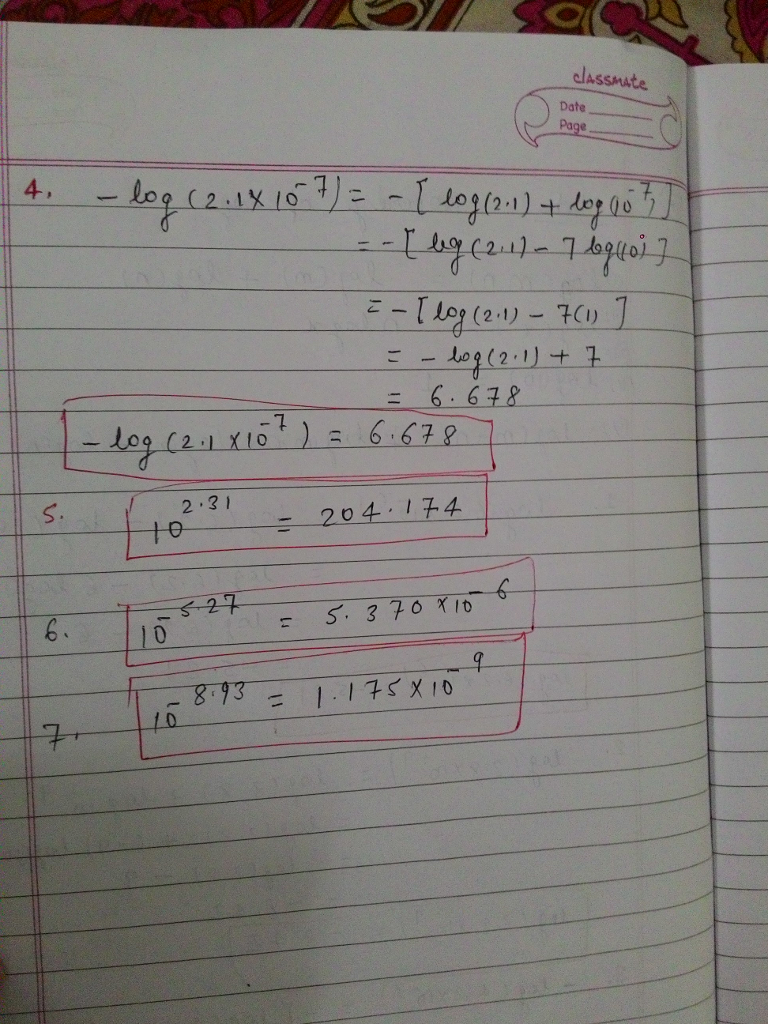# Homework Solution: Give numerical answers for the following log and anti-log problems log 6.2 times…Give numerical answers for the following log and anti-log problems log 6.2 times 10^-6 = _____ log 3.8 times 10^-9 = _____ -log 4.2 times 10^-3 = ____ -log 2.1 times 10^-7 = _____ 10^2.31 = ____ 10^-5.27 = ____ 10^-8.93 = _____